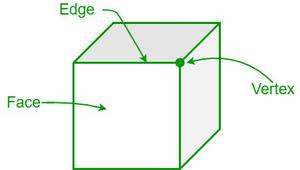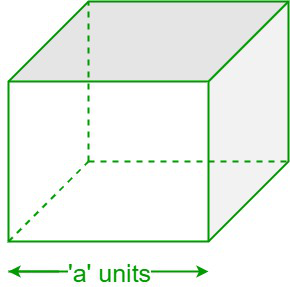# How to find the Surface Area of a Cube?

• Last Updated : 12 May, 2022

In geometry, a cube is a solid three-dimensional form of a square. A cube has six square faces, eight vertices, and twelve edges. A Rubik’s cube, sugar cubes, an ice cube, dice, etc., are some examples of the cube. The faces of a cube share a common boundary called the edge. The edge of a cube depicts the length, breadth, and height of a cube, which are connected at the vertex. Since the six faces of a cube are squares, the length, breadth, and height of a cube are equal. A cube is one of the five platonic solids and is also known as an equilateral cuboid, a square parallelepiped, or a right rhombic hexahedron. As all the sides of a cube are square-shaped, a cube is a particular case of a square prism.Cube

Surface Area of a Cube

In geometry, the region occupied by any shape is called the area. The total area covered by all six sides or faces of a cube is called the surface area. Hence, the total surface area of a cube is the sum of the areas of its six faces or sides. The total surface area of a cube is equal to six times the square length of the sides of a cube, i.e., 6a2, where a is the length of the edge of a cube.Cube

Let the length of the edge of a cube be “a” unit. Since each face of a cube is a square, the area of each face of the cube is equal to the area of a square, i.e., a2. As a cube consists of 6 faces,

The total surface of the cube = Sum of the areas of six square faces of the cube

= a2 + a2 + a2 + a2 + a2 + a2 = 6a2

Hence, the total surface area of a cube (TSA) = 6a2

The total surface area of a cube (TSA) = 6a2 square units

The lateral surface area of a Cube

The lateral surface area of a cube is the sum of the areas of all its faces, except its top and bottom faces.

Hence, the lateral surface area of the cube (LSA) = the sum of areas of all four side faces of a cube

= a2 + a2 + a2 + a2 = 4a2

The lateral surface area of the cube (LSA) = 4a2 square units

### Sample problems

Problem 1: What is the total surface area of the cube if its side is 6 cm?

Solution:

Given, Side of the cube = 6 cm

The total surface area of the cube = 6a2

= 6 × 62 cm2

= 6 × 36 cm2 = 216 cm2

Hence, the surface area of the cube is 216 cm2.

Problem 2: Find the side of a cube whose total surface area is 1350 cm2.

Solution:

Given, Surface area of the cube = 1350 cm2

Let the side of the cube be “a” cm.

We know that the surface area of the cube = 6a2

⇒ 6a2 = 1350

⇒ a2 = 1350/6 = 225

⇒ a = √225 = 15 cm

Hence, the side of the cube = 15 cm.

Problem 3: The length of the side of the cube is 10 inches. Find the lateral surface and total surface areas of a cube?

Solution:

Given, the length of the side = 10 in

We know,

The lateral surface area of a cube = 4a2

= 4 × (10)2

= 4 × 100 = 400 square inches

The total surface of a cube = 6a2

= 6 × (10)2

= 6 × 100 = 600 square inches.

Therefore, the lateral surface area of a cube is 400 square inches and its total surface area is 600 square inches.

Problem 4: John is playing with a Rubik’s cube whose base area is 16 square inches. What is the length of the side of a cube, and what is its lateral surface area?

Solution:

Given, the base area of the cube = 16 square inches

Let the length of the side of a cube be “a” inches.

We know,

The base area of a cube = a2 = 16

⇒ a = √16 = 4 inches

The lateral surface of a cube = 4a2

= 4 × 42

= 4 × 16 = 64 square inches

Hence, the length of the side of the cube is 4 inches and its lateral surface area is 64 square inches.

Problem 5: A cubical container with a side of 5 meters is to be painted on the entire outer surface area. Find the area to be painted and the total cost of painting the cube at a rate of ₨ 30 per square meter.

Solution:

Given, the length of the cubical container = 5 m

Since the area to be painted is on the outer surface, the area to be painted is equal to the total surface area of the cubical container. Hence, we need to find the total surface area of the cubical container.

Therefore, the total surface of the cubical container = 6 × (side)2

= 6 × (5)2

= 6 × 25

= 150 square meters.

Given, the cost of painting per 1 square meter = ₨ 30

Hence, the total cost of painting = ₨ (150 × 30) = ₨ 4500/-

Problem 6: Find the ratio of the total surface area of a cube to its lateral surface area?

Solution:

Let the length of the side of a cube be “s” units.

The total surface area of the cube (TSA) = 6s2

The lateral surface area of the cube (LSA) = 4s2

Now, the ratio of the total surface area of a cube to its lateral surface area = TSA/LSA

⇒ TSA/LSA = 6s2/4s2 = 3/2

Therefore, the ratio of the total surface area of a cube to its lateral surface area is 3 : 2.

My Personal Notes arrow_drop_up
Recommended Articles
Page :